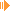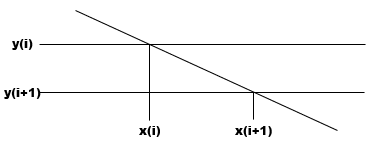Polygon Filling (with Scanline)

Updated July 2010 (Version 1.1) : Fixed bug to handle horiziontal lines correctly.Download Applet with Source (Version 1.1, 25kb)

Drawing a polygon is trivial, but what about filling the area of an (arbitrary) polygon? Hm, that's more interesting. So searching the net, I came often across the Flood Fill Algorithm, but there you need a point inside the polygon to begin and it has also other disadvantages (e.g with 'complicated' polygons).
Quite cooler is the Scanline Algorithm - there you move a horizontal line step by step over the polygon and calculate the intersection points with the polygon.

The main steps are:Sorting the polygon edges ascendend by their y-coordinate (so from smallest to biggest one).Move the scanline step by step from the smallest to the biggest y-coordinatefor each scanline position do:
- get all edges which are currently intersected by the scanline
- calculate the intersection points and sort them ascending by their x-coordinate
- draw the line segments inside the polygon area

For example look at this polygon:The sequence of edges sorted by their smallest y-coordinate (assume the y-axis goes from top to bottom) would be 1234567. The edges intersected by the scanline (the so-called active edges) are 3654. Calculating these four intersections and sorting them by their x-coordinates give two line segments 3-6 and 5-4 which can easily be drawn. Afterwards the scanline would be shifted down one pixel etc.

The sorting of the edges is not explicitly necessary: for each scanline position you could try to intersect it with all polygon edges which is not that effective. The sorting allows us to easily keep a list of active edges:
If the scanline moves over the endpoint of an edge with the smaller y-coordinate it is added to the list; if the scanline moves over the endpoint of an edge with the bigger y-coordinate it is deleted from the list.

This also allows an simpler way of calculating the intersection points. Look at this:Cause you know your active edges, you can get the intersection point of an edge with scanline y(i+1) from its previous intersection point with scanline y(i).

 Slope m is: m = (y(i) - y(i+1)) / (x(i) - x(i-1)). Cause we have y(i) - y(i+1) = 1, we get: x(i+1) = x(i) - 1/m.

There are also some special cases you have to consider:
Depending on your implementation it could be better to ignore horizontal lines cause they does not have to be drawn and also have many intersection points with the scanline which can lead to problems.
Also to get the correct line segments when the scanline hits an endpoint of an edge, look at these 3 cases:If both edges are above or beneath the scanline, it could be better to count the intersection twice; while if one edge is beneath and one above it's better to count the intersection point just once.

I think this is enough information for coding your own polygon filling algorithm. Below you can play around with my implementation. But note in case you're looking at the code that my implementation differs in some small things (like I calc x(i+1) = x(i) + 1/m instead of x(i+1) = x(i) - 1/m and so on). So don't get confused and enjoy it!

This site is part of Sunshine's Homepage